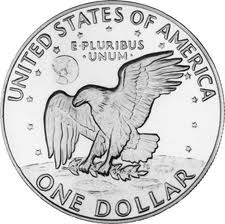# Probabilities

One cannot discern outcomes in all situations, for example whether we will get heads or tails when tossing a coin. We cannot foresee how the coin will land as it is decided by chance. However, if we were to toss the coin infinite times, then half of the results will be heads and half will be tails.One may say that the probability of achieving heads is 0.5 and the probability for tails is 0.5.

One designates probability by the letter P, and writes the probability of achieving heads as: P(heads)=0,5 and tails as: P(tails)=0,5 for tails.

The probability of an event always lies between 0 (0%) and 1 (100%) and is calculated by the following formula:

$Probability=\frac{number \; of\; favorable\; outcomes}{number \;of\; possible\; outcomes}$

Example

What is the probability of throwing a 5 with a die?

The number of favorable outcomes = 1 (there is only one 5 on a die)

The number of possible outcomes = 6 (a die has 6 sides)

$P(5)=\frac{1}{6}\approx 0.167$

In order to calculate this probability one multiplies the probability of the one event with that of the other one:

$P(A\;and\;B)=P(A)\cdot P(B)$

Example

If one has two dice, what is the probability of throwing a 5 with the first die and a 6 with the other die?

P(5)=1/6

P(6)=1/6

$P(5\;and\;6)=P(5)\cdot P(6)=\frac{1}{6}\cdot \frac{1}{6}=\frac{1}{36}$

In order to add two probabilities we have to determine whether or not they are mutually exclusive or inclusive events. Our events are considered to be mutually exclusive if they cannot happen at the same time.

Example

We toss a coin, either heads or tails might turn up, but not heads and tails at the same time.

If A and B are mutually exclusive events then we determine the probability of A happening or the probability of B happening with the following formula:

$P(A\;or\;B )=P(A)+P(B)$

If the events are inclusive, e.g. both can happen but not at the same time, then we use the following formula to determine the probability that either A or B occurs:

$P(A\;or\;B )=P(A)+P(B)-P(A\; and\; B)$

## Video lesson

What is the probability of not throwing a 6 when throwing a die?